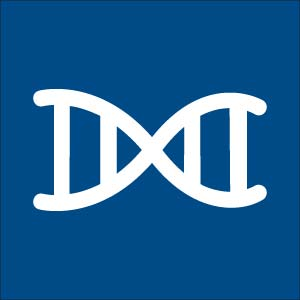# Calculations: Converting from nanograms to copy numberHere is a calculation often used when creating a qPCR standard curve. Link to a free, online tool that will do it for you.

Researchers performing qPCR will often create a standard curve based on nanograms of amplicon, and then need to convert the resulting nanograms detected to copy number. The formula for making this conversion is as follows:

Where: X = amount of amplicon (ng)
N = length of dsDNA amplicon
660 g/mole = average mass of 1 bp dsDNA

† The actual oligonucleotide MW (in Daltons or g/mole) is provided on the IDT Spec Sheet for each oligonucleotide and can be substituted for this arithmetic phrase, which only provides an average MW for a sequence of this length.

An alternative is to create the standard curve based on copy number; e.g., ranging from 102 to 107 copies, in 10-fold increments. You can then determine copy number directly from the standard curve.

### Let free software do it for you!

A convenient, free-access copy number calculator was developed by former IDT customer, Andrew Staroscik, who has since left the bench. The calculator can be found at: www.scienceprimer.com/copy-number-calculator-for-realtime-pcr.

Published Oct 21, 2013
Revised/updated Mar 16, 2017